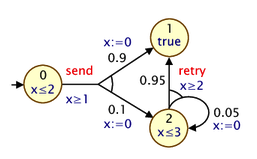# Real-time Models

So far in this section, we have mainly focused on three types of models: DTMCs, MDPs and CTMCs, in which all the variables making up their state are finite. PRISM also supports real-time models, in particular, probabilistic timed automata (PTAs), which extend MDPs with the ability to model real-time behaviour. This is done in the style of timed automata [AD94], by adding clocks, real-valued variables which increase with time and can be reset. For background material on PTAs, see for example [NPS13]. You can also find several example PTA models included in the PRISM distribution. Look in the `prism-examples/ptas` directory.

Before describing how PTA features are incorporated into the PRISM modelling language, we give a simple example. Here is a small PTA:and here is a corresponding PRISM model:

pta

module M

s : [0..2] init 0;
x : clock;

invariant
(s=0 => x<=2) &
(s=2 => x<=3)
endinvariant

[send] s=0 & x>=1 -> 0.9:(s'=1)&(x'=0) + 0.1:(s'=2)&(x'=0);
[retry] s=2 & x>=2 -> 0.95:(s'=1) + 0.05:(s'=2)&(x'=0);

endmodule

For modelling PTAs in PRISM, there is a new datatype, `clock`, used for variables that are clocks. These must be local to a particular module, not global. Other types of PRISM variables can be defined in the usual way. In the example above, we use just a single integer variable `s` to represent the locations of the PTAs.

In a PTA, transitions can include a guard, which constrains when it can occur based on the current value of clocks, and resets, which specify that a clock's values should be set to a new (integer) value. These are both specified in PRISM commands in the usual way: see, for example, the inclusion of `x>=1` in the guard for the `send`-labelled command and the updates of the form `(x'=0)` which reset the clock `x` to 0.

The other new addition is an `invariant` construct, which is used to specify an expression describing the clock invariants for each PRISM module. These impose restrictions on the allowable values of clock variables, depending on the values of the other non-clock variables. The `invariant` construct should appear between the variable declarations and the commands of the module. Often, clock invariants are described separately for each PTA location; hence, the invariant will often take the form of a conjunction of implications, as in the example model above, but more general expressions are also permitted. In the example, the clock `x` must satisfy `x<=2` or `x<=3` when local variables `s` is 0 or 2, respectively. If `s` is 1, there is no restriction (since the invariant is effectively `true` in this case).

Expressions that include reference to clocks, whether in guards or invariants, must satisfy certain conditions to facilitate model checking. In particular, references to clocks must appear as conjunctions of simple clock constraints, i.e. conjunctions of expressions of the form `x~c` or `x~y` where `x` and `y` are clocks, `c` is an integer-valued expression and `~` is one of `<`, `<=`, `>=`, `>`, `=`).

There are also some additional restrictions imposed on PTA models that are dependent on which of the PTA model checking engines is in use.

For the stochastic games and backwards reachability engines:

• Modules cannot read the local variables of other modules and global variables are not permitted.
• The model must also have a single initial state (i.e. the `init...endinit` construct is not permitted).

For the digital clocks engine:

• Clock constraints cannot use strict comparison operators, e.g. `x<=5` is allowed, but `x<5` is not.
• Diagonal clock constraints are not allowed, i.e. those containing references to two clocks, such as `x<=y`.

Finally, PRISM makes several assumptions about PTAs, regardless of the engine used.

• Firstly PTAs should not exhibit timelocks, i.e. the possibility of reaching a state where no transitions are possible and time cannot elapse beyond a certain point (due to invariant conditions). PRISM checks for timelocks and reports an error if one is found.
• Secondly, PTAs should be well-formed and non-zeno (see e.g. [KNSW07] for details). Currently, PRISM does not check automatically that these assumptions are satisfied.